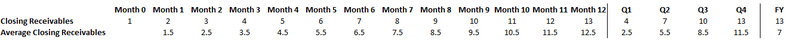# Calculating the average of a balance sheet item for quarterly / annual totalsI have a number of balance sheet accounts (e.g. Closing Receivables*) for which I need to calculate the average value.

This should be calculated as follows:

- Monthly: average between the current month and prior month value

- Quarterly Total: average of the last 4 data points

- Annual Total: average of the last 13 data points

*note that Closing Receivables will have 'Sum, Time: Closing Balance' as its aggregation setting

Below is a worked example:Currently my method to do this seems complex:

- Create an 'Opening Receivables' line item with calculation 'previous(Closing Receivables)' and aggregation 'Sum, Time: Opening Balance'

- Create a 'Closing Receivables (time sum)' line item with calculation 'Closing Receivables' and aggregation 'Sum, Time: Sum'

- Create a 'Time Aggregation' line item with calculation '1' and aggregation 'Average, Time: Sum'

Then apply the following calculation to the Average Receivables line item with aggregation 'Formula':

(Opening Receivables + 'Closing Receivables (Time Sum)') / (Time Aggregation + 1)

I am hoping that someone can suggest a simpler way to achieve this? My particular concern is that for every closing balance that I need an average for, I will need to create 2 additional line items to feed into the average calc (the Time Aggregation line item can be shared).

Tagged: